## Getting Started: TCOUNTREG Procedure

The TCOUNTREG procedure is similar in use to other regression model procedures in the SAS System. For example, the following statements are used to estimate a Poisson regression model:

   proc tcountreg data=one ;
model y = x / dist=poisson ;
run;


The response variable
is numeric and has nonnegative integer values. To allow for variance greater than the mean, specify the DIST=NEGBIN option to fit the negative binomial model instead of the Poisson.

The following example illustrates the use of PROC TCOUNTREG. The data are taken from Long (1997) and can be found in the SAS/ETS Sample Library. This study examines how factors such as gender (fem), marital status (mar), number of young children (kid5), prestige of the graduate program (phd), and number of articles published by a scientist’s mentor (ment) affect the number of articles (art) published by the scientist.

The first 10 observations are shown in Figure 30.1.

Figure 30.1: Article Count Data

Obs art fem mar kid5 phd ment
1 3 0 1 2 1.38000 8.0000
2 0 0 0 0 4.29000 7.0000
3 4 0 0 0 3.85000 47.0000
4 1 0 1 1 3.59000 19.0000
5 1 0 1 0 1.81000 0.0000
6 1 0 1 1 3.59000 6.0000
7 0 0 1 1 2.12000 10.0000
8 0 0 1 0 4.29000 2.0000
9 3 0 1 2 2.58000 2.0000
10 3 0 1 1 1.80000 4.0000

The following SAS statements estimate the Poisson regression model:

proc tcountreg data=long97data;
model art = fem mar kid5 phd ment / dist=poisson;
run;


The Model Fit Summary table, shown in Figure 30.2, lists several details about the model. By default, the TCOUNTREG procedure uses the Newton-Raphson optimization technique. The maximum log-likelihood value is shown, in addition to two information measures, Akaike’s information criterion (AIC) and Schwarz’s Bayesian information criterion (SBC), which can be used to compare competing Poisson models. Smaller values of these criteria indicate better models.

Figure 30.2: Estimation Summary Table for a Poisson Regression

The TCOUNTREG Procedure

Model Fit Summary
Dependent Variable art
Number of Observations 915
Data Set WORK.LONG97DATA
Model Poisson
Optimization Method Newton-Raphson
Log Likelihood -1651
Number of Iterations 5
AIC 3314
SBC 3343

The parameter estimates of the model and their standard errors are shown in Figure 30.3. All covariates are significant predictors of the number of articles, except for the prestige of the program (phd), which has a
-value of 0.6271.

Figure 30.3: Parameter Estimates of Poisson Regression

Parameter Estimates
Parameter DF Estimate Standard Error t Value Approx
Pr > |t|
Intercept 1 0.304617 0.102982 2.96 0.0031
fem 1 -0.224594 0.054614 -4.11 <.0001
mar 1 0.155243 0.061375 2.53 0.0114
kid5 1 -0.184883 0.040127 -4.61 <.0001
phd 1 0.012823 0.026397 0.49 0.6271
ment 1 0.025543 0.002006 12.73 <.0001

The following statements fit the negative binomial model. Although the Poisson model requires that the conditional mean and conditional variance be equal, the negative binomial model allows for overdispersion; that is, the conditional variance can exceed the conditional mean.

proc tcountreg data=long97data;
model art = fem mar kid5 phd ment / dist=negbin(p=2) method=qn;
run;


The fit summary is shown in Figure 30.4, and parameter estimates are listed in Figure 30.5.

Figure 30.4: Estimation Summary Table for a Negative Binomial Regression

The TCOUNTREG Procedure

Model Fit Summary
Dependent Variable art
Number of Observations 915
Data Set WORK.LONG97DATA
Model NegBin
Optimization Method Quasi-Newton
Log Likelihood -1561
Number of Iterations 16
AIC 3136
SBC 3170

Figure 30.5: Parameter Estimates of Negative Binomial Regression

Parameter Estimates
Parameter DF Estimate Standard Error t Value Approx
Pr > |t|
Intercept 1 0.256144 0.138560 1.85 0.0645
fem 1 -0.216418 0.072672 -2.98 0.0029
mar 1 0.150489 0.082106 1.83 0.0668
kid5 1 -0.176415 0.053060 -3.32 0.0009
phd 1 0.015271 0.036040 0.42 0.6718
ment 1 0.029082 0.003470 8.38 <.0001
_Alpha 1 0.441620 0.052967 8.34 <.0001

The parameter estimate for _Alpha of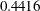is an estimate of the dispersion parameter in the negative binomial distribution. Atest for the hypothesis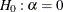is provided. It is highly significant, indicating overdispersion (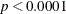).

The null hypothesiscan be also tested against the alternative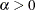by using the likelihood ratio test, as described by Cameron and Trivedi (1998, pp. 45, 77–78). The likelihood ratio test statistic is equal to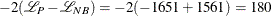, where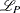and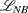are the log likelihoods for the Poisson and negative binomial models, respectively. The likelihood ratio test is highly significant, providing strong evidence of overdispersion.